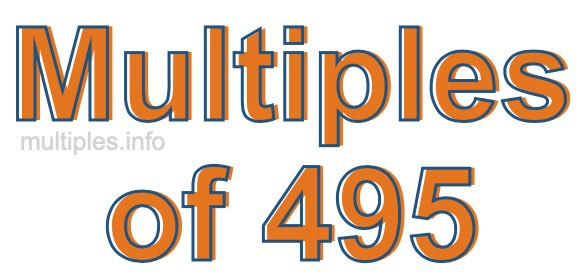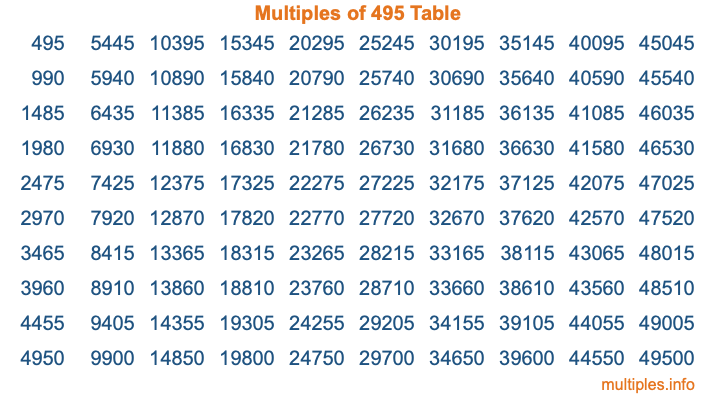Multiples of 495Welcome to the Multiples of 495 page. Here we will first teach you everything you will ever need to know about the multiples of 495, and then give you a study guide summary of everything we taught you to make sure you remember it all. Use this page to look up facts and learn information about the multiples of 495. This page will make you a multiples of four hundred ninety-five expert!

Definition of Multiples of 495
Multiples of 495 are all the numbers that when divided by 495 equal an integer. Each of the multiples of 495 are called a multiple. A multiple of 495 is created by multiplying 495 by an integer.

Therefore, to create a list of multiples of 495, you start with 1 multiplied by 495, then 2 multiplied by 495, then 3 multiplied by 495, and so on for as long as you want. Thus, the list of the first five multiples of 495 is 495, 990, 1485, 1980, and 2475. To see a larger list of multiples of 495, see the printable image of Multiples of 495 further down on this page. We also have a category where you can choose any nth multiple of 495.

Multiples of 495 Checker
The Multiples of 495 Checker below checks to see if any number of your choice is a multiple of 495. In other words, it checks to see if there is any number (integer) that when multiplied by 495 will equal your number. To do that, we divide your number by 495. If the the quotient is an integer, then your number is a multiple of 495.

Is  a multiple of 495?

Least Common Multiple of 495 and ...
A Least Common Multiple (LCM) is the lowest multiple that two or more numbers have in common. This is also called the smallest common multiple or lowest common multiple and is useful to know when you are adding our subtracting fractions. Enter one or more numbers below (495 is already entered) to find the LCM.

Check out our LCM Calculator if you need more details about the Least Common Multiple or if you need the LCM for different numbers for adding and subtraction fractions.

nth Multiple of 495
As we stated above, 495 is the first multiple of 495, 990 is the second multiple of 495, 1485 is the third multiple of 495, and so on. Enter a number below to find the nth multiple of 495.

th multiple of 495

Multiples of 495 vs Factors of 495
495 is a multiple of 495 and a factor of 495, but that is where the similarities end. All postive multiples of 495 are 495 or greater than 495. All positive factors of 495 are 495 or less than 495.

Below is the beginning list of multiples of 495 and the factors of 495 so you can compare:

Multiples of 495: 495, 990, 1485, 1980, 2475, etc.

Factors of 495: 1, 3, 5, 9, 11, 15, 33, 45, 55, 99, 165, 495

As you can see, the multiples of 495 are all the numbers that you can divide by 495 to get a whole number. The factors of 495, on the other hand, are all the whole numbers that you can multiply by another whole number to get 495.

It's also interesting to note that if a number (x) is a factor of 495, then 495 will also be a multiple of that number (x).

Multiples of 495 vs Divisors of 495
The divisors of 495 are all the integers that 495 can be divided by evenly. Below is a list of the divisors of 495.

Divisors of 495: 1, 3, 5, 9, 11, 15, 33, 45, 55, 99, 165, 495

The interesting thing to note here is that if you take any multiple of 495 and divide it by a divisor of 495, you will see that the quotient is an integer.

Multiples of 495 Table
Below is an image of the first 100 multiples of 495 in a table. The table is in chronological order, column by column. The first column has the first ten multiples of 495, the second column has the next ten multiples of 495, and so on.The Multiples of 495 Table is also referred to as the 495 Times Table or Times Table of 495. You are welcome to print out our table for your studies.

Negative Multiples of 495
Although not often discussed or needed in math, it is worth mentioning that you can make a list of negative multiples of 495 by multiplying 495 by -1, then by -2, then by -3, and so on, to get the following list of negative multiples of 495:

-495, -990, -1485, -1980, -2475, etc.

Multiples of 495 Summary
Below is a summary of important Multiples of 495 facts that we have discussed on this page. To retain the knowledge on this page, we recommend that you read through the summary and explain to yourself or a study partner why they hold true.

There are an infinite number of multiples of 495.

A multiple of 495 divided by 495 will equal a whole number.

495 divided by a factor of 495 equals a divisor of 495.

The nth multiple of 495 is n times 495.

The largest factor of 495 is equal to the first positive multiple of 495.

495 is a multiple of every factor of 495.

495 is a multiple of 495.

A multiple of 495 divided by a divisor of 495 equals an integer.

495 divided by a divisor of 495 equals a factor of 495.

Any integer times 495 will equal a multiple of 495.

Multiples of a Number
Here you can get the multiples of another number, all with the same attention to detail as we did for multiples of 495 on this page.

Multiples of
Multiples of 496
Did you find our page about multiples of four hundred ninety-five educational? Do you want more knowledge? Check out the multiples of the next number on our list!